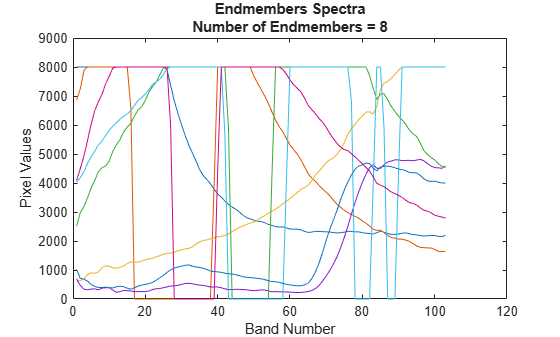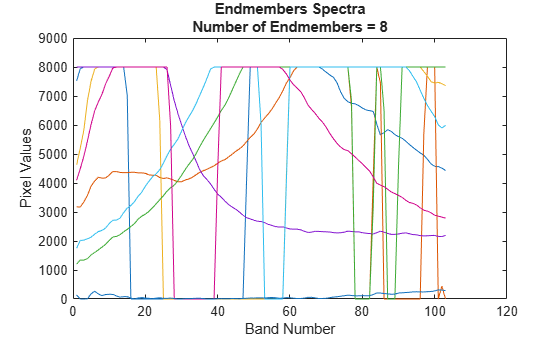# nfindr

Extract endmember signatures using N-FINDR

## Syntax

``endmembers = nfindr(inputData,numEndmembers)``
``endmembers = nfindr(inputData,numEndmembers,Name,Value)``

## Description

example

````endmembers = nfindr(inputData,numEndmembers)` extracts endmember signatures from hyperspectral data `cube` by using the N-finder (N-FINDR) algorithm. `numEndmembers` is the number of endmember signatures to be extracted using N-FINDR algorithm. For more information about the N-FINDR method, see Algorithms.```

example

````endmembers = nfindr(inputData,numEndmembers,Name,Value)` specifies options using one or more name-value pair arguments in addition to the input arguments in the previous syntax. Use this syntax to set the options for number of iterations and dimensionality reduction. NoteThis function requires the Image Processing Toolbox™ Hyperspectral Imaging Library. You can install the Image Processing Toolbox Hyperspectral Imaging Library from Add-On Explorer. For more information about installing add-ons, see Get and Manage Add-Ons. ```

## Examples

collapse all

Read a hyperspectral data into the workspace.

`hcube = hypercube('paviaU.hdr');`

Find the number of spectrally distinct endmembers present in the hyperspectral data cube by using `countEndmembersHFC` function.

`numEndmembers = countEndmembersHFC(hcube,'PFA',10^-7);`

Compute the endmembers using the N-FINDR method. By default, the `nfindr` function uses maximum noise fraction (MNF) transform for preprocessing. The default value for number of iterations is 3 times the number of estimated endmembers.

`endmembers = nfindr(hcube.DataCube,numEndmembers);`

Plot the endmembers of the hyperspectral data.

```figure plot(endmembers) xlabel('Band Number') ylabel('Pixel Values') ylim([0 9000]) title({'Endmembers Spectra',['Number of Endmembers = ' num2str(numEndmembers)]});```Read a hyperspectral data into the workspace.

`hcube = hypercube('paviaU.hdr');`

Find the number of spectrally distinct endmembers present in the hyperspectral data cube by using `countEndmembersHFC` function.

`numEndmembers = countEndmembersHFC(hcube,'PFA',10^-7);`

Compute the endmembers using the N-FINDR method. Specify the value for number of iterations as 1000. Select principal component analysis (PCA) as the dimensionality reduction method for preprocessing.

`endmembers = nfindr(hcube.DataCube,numEndmembers,'NumIterations',1000,'ReductionMethod','PCA');`

Plot the endmembers of the hyperspectral data.

```figure plot(endmembers) xlabel('Band Number') ylabel('Pixel Values') ylim([0 9000]) title({'Endmembers Spectra',['Number of Endmembers = ' num2str(numEndmembers)]});```## Input Arguments

collapse all

Input hyperspectral data, specified as an 3-D numeric array or a `hypercube` object. If the input is a `hypercube` object, then the function reads the hyperspectral data from its `DataCube` property.

The hyperspectral data is an numeric array of size M-by-N-by-C. M and N are the number of rows and columns in the hyperspectral data respectively. C is the number of spectral bands in the hyperspectral data.

Data Types: `single` | `double` | `int8` | `int16` | `int32` | `int64` | `uint8` | `uint16` | `uint32` | `uint64`

Number of endmembers to extract, specified as a positive scalar integer. The value must be in the range [`1` C]. C is the number of spectral bands in the input hyperspectral data.

Data Types: `single` | `double` | `int8` | `int16` | `int32` | `int64` | `uint8` | `uint16` | `uint32` | `uint64`

### Name-Value Arguments

Specify optional comma-separated pairs of `Name,Value` arguments. `Name` is the argument name and `Value` is the corresponding value. `Name` must appear inside quotes. You can specify several name and value pair arguments in any order as `Name1,Value1,...,NameN,ValueN`.

Example: `nfindr(cube,7,'NumIterations',100,'Method','None')`

Number of iterations, specified as a positive scalar integer. The default value is `3P`. P is the number of endmember signatures to be extracted. The computation time of the algorithm increases with the increase in the number of iterations.

Data Types: `single` | `double` | `int8` | `int16` | `int32` | `int64` | `uint8` | `uint16` | `uint32` | `uint64`

Dimensionality reduction method, specified as one of these values:

• `'MNF'` — To perform dimensionality reduction by using the maximum noise fraction (MNF) method. This is the default.

• `'PCA'` — To perform dimensionality reduction by using the principal component analysis (PCA) method.

If you specify this argument, the function first reduces the spectral dimension of the input data by using the specified method. Then, it computes the endmember signatures from the reduced data.

Data Types: `char` | `string`

## Output Arguments

collapse all

Endmember signatures, returned as a matrix of size C-by-P and datatype same as the datatype of the input hyperspectral data.

Data Types: `single` | `double` | `int8` | `int16` | `int32` | `int64` | `uint8` | `uint16` | `uint32` | `uint64`

## Algorithms

N-FINDR is an iterative approach for finding the endmembers of a hyperspectral data. The method assumes that the volume of a simplex formed by the endmembers (purest pixels) is larger than any other volume defined by any other combination of pixels . The steps involved are as follows:

1. Compute principal component bands and reduce the spectral dimensionality of the input data by using MNF or PCA. The number of principal component bands to be extracted is set equal to the number of endmembers to be extracted. The endmembers are extracted from the principal component bands.

2. Randomly select n number of pixel spectra from the reduced data as initial set of endmembers.

3. For iteration 1, denote the initial set of endmembers as $\text{\hspace{0.17em}}\left\{{\text{e}}_{1}^{\left(1\right)},\text{\hspace{0.17em}}\text{\hspace{0.17em}}{\text{e}}_{2}^{\left(1\right)},\text{\hspace{0.17em}}\text{\hspace{0.17em}}\cdots \text{\hspace{0.17em}},\text{\hspace{0.17em}}\text{\hspace{0.17em}}{\text{e}}_{p}^{\left(1\right)}\right\}$.

Consider the endmembers as vertices of a simplex and compute the volume by using

`$\text{\hspace{0.17em}}V\left({\text{E}}^{\left(1\right)}\right)\text{\hspace{0.17em}}=\text{\hspace{0.17em}}|\mathrm{det}\left({\text{E}}^{\left(1\right)}\right)|$`

where ${\text{E}}^{\left(1\right)}\text{\hspace{0.17em}}=\text{\hspace{0.17em}}\text{\hspace{0.17em}}\left[\begin{array}{l}1\text{\hspace{0.17em}}\text{\hspace{0.17em}}\text{\hspace{0.17em}}\text{\hspace{0.17em}}\text{\hspace{0.17em}}\text{\hspace{0.17em}}\text{\hspace{0.17em}}\text{\hspace{0.17em}}\text{\hspace{0.17em}}\text{\hspace{0.17em}}\text{\hspace{0.17em}}\text{\hspace{0.17em}}1\text{\hspace{0.17em}}\text{\hspace{0.17em}}\text{\hspace{0.17em}}\text{\hspace{0.17em}}\text{\hspace{0.17em}}\text{\hspace{0.17em}}\text{\hspace{0.17em}}\text{\hspace{0.17em}}\cdots \text{\hspace{0.17em}}\text{\hspace{0.17em}}\text{\hspace{0.17em}}\text{\hspace{0.17em}}\text{\hspace{0.17em}}\text{\hspace{0.17em}}\text{\hspace{0.17em}}1\\ {\text{e}}_{1}^{\left(1\right)}\text{\hspace{0.17em}}\text{\hspace{0.17em}}\text{\hspace{0.17em}}\text{\hspace{0.17em}}{\text{e}}_{2}^{\left(1\right)}\text{\hspace{0.17em}}\text{\hspace{0.17em}}\text{\hspace{0.17em}}\text{\hspace{0.17em}}\cdots \text{\hspace{0.17em}}\text{\hspace{0.17em}}\text{\hspace{0.17em}}\text{\hspace{0.17em}}{\text{e}}_{p}^{\left(1\right)}\end{array}\right]\text{\hspace{0.17em}}\text{\hspace{0.17em}}\text{\hspace{0.17em}}$.

4. For iteration 2, Select a new pixel spectra r, such that $\text{\hspace{0.17em}}\text{r}\text{\hspace{0.17em}}\notin \left\{{\text{e}}_{1}^{\left(1\right)},\text{\hspace{0.17em}}\text{\hspace{0.17em}}{\text{e}}_{2}^{\left(1\right)},\text{\hspace{0.17em}}\text{\hspace{0.17em}}\cdots \text{\hspace{0.17em}},\text{\hspace{0.17em}}\text{\hspace{0.17em}}{\text{e}}_{p}^{\left(1\right)}\right\}$.

5. Replace each endmember in the set with r and compute the volume of the simplex V(E(2)).

6. Replace the ith endmember in the set with r, if the computed volume V(E(2)) is greater than V(E(1)). This results in an updated set of endmembers. For example, if i = 2, the new set of endmembers derived at the end of the second iteration is $\text{\hspace{0.17em}}\left\{{\text{e}}_{1}^{\left(2\right)},\text{\hspace{0.17em}}\text{\hspace{0.17em}}{\text{e}}_{2}^{\left(2\right)}=\text{\hspace{0.17em}}\text{\hspace{0.17em}}\text{r},\text{\hspace{0.17em}}\text{\hspace{0.17em}}\cdots \text{\hspace{0.17em}},\text{\hspace{0.17em}}\text{\hspace{0.17em}}{\text{e}}_{p}^{\left(2\right)}\right\}$.

7. For each iteration, select a new pixel spectra r and repeat steps 5 and 6. Each iteration results in an update set of endmembers. The iteration ends when the total number of iterations reaches the specified value `NumIterations`.

 Winter, Michael E. “N-FINDR: An Algorithm for Fast Autonomous Spectral End-Member Determination in Hyperspectral Data.” Proc. SPIE Imaging Spectrometry V 3753, (October 1999): 266–75. https://doi.org/10.1117/12.366289.